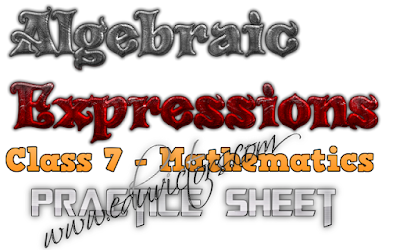## Pages

Showing posts with label class7-maths. Show all posts
Showing posts with label class7-maths. Show all posts

## Tuesday, 3 May 2022

For Class 7 / Class 8 / Class 9

The worksheet will help you understand the basic definition of Rational and its properties.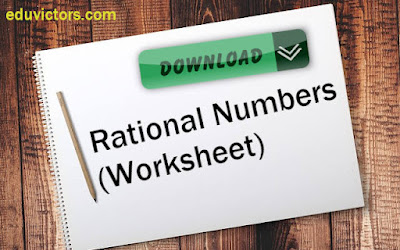# Class 7 Maths - Ratio and Proportion (Worksheet - Solved)

Worksheet

Q1: In ratio a : b, a is called as _______ or ________.

Q2: In ratio a : b, b is called as _______ or ________.

Q3: Express 75 : 125 in its simplest term.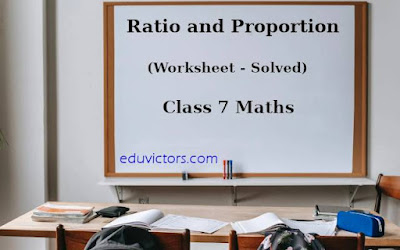# CBSE Class 7 - Maths - Solved Problems on Direct Variation

If two varying quantities are always in the same ratio, they are said to vary directlt or are directly proportional to one another. Or we simpley say that they are proportional.

In direct variation, as one quantity increases, the other quatity also increases.

If a and b are the two quantities in direct variation then, a ∝ b

e.g. At constant speed, distance covered varies directly as time, i.e., the longer the time an objec travels, the greater the distance it will cover.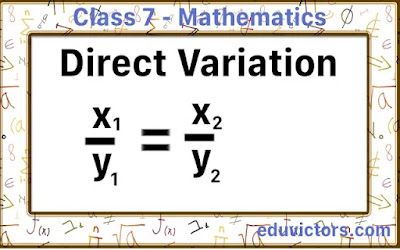# National Mathematics Day

India celebrates National Mathematics Day on December 22 every year. The day marks the birth anniversary of famous mathematician Srinivasa Ramanujan.

*Typo in the graphic new = knew# CBSE Class 7 - Maths - Fractions - Solved Problems

A fraction is a part of whole.

Fractions are of various types. Commonly used fractions are mixed fractions, proper fractions

e.g. $\frac{13}{4}, 2\frac{3}{4}, \frac{1}{2}$ etc.

COMPARE FRACTIONS

We can compare two or more fractions by taking the L.C.M of the denominators of the given fractions. Fraction with a greater denominator is bigger and vice versa.

Q1: Compare $\frac{23}{52} \textrm{ and } \frac{19}{22}$

Answer: $\frac{23}{52} > \frac{19}{22}$# Class 7 Maths Annual Sample Question Paper  (Set-1) 2020-21

Learning is a continual process. To improve scores, the best way is to learn and practice. Solving question papers help students to evaluate their knowledge and familarise with different types of question patterns being asked in examination.

Eduvictors provides CBSE Previous Year Papers and Sample Question Papers for Class 7 Maths, Science, Social, English, Hindi and Sanskrit with/without solutions to help students in their board exam preparation.

Here attached the Maths Sample Question Paper (2020-2021).## Chapter 9 - Rational Numbers (Worksheet)

CBSE Class 7 Maths

Fill in the blanks

1. A rational number is a number that can be written in the form p/q, where p and q are ________ and q ____ 0.

2. The ________ of a rational number can never be zero.

3. The rational number _______ is neither negative nor positive.## Tuesday, 30 July 2019

### CBSE Class 7 Maths - Lines and Angles - Worksheet -2 (#eduvictors)(#cbsenotes)

Lines and Angles (Worksheet -2)
CBSE Class 7 Maths

Fill in the blanks

1. A/An _________ is formed when lines or line segments meet.

2. The lines that form an angle are called the _______ or the _______ of the angle.

3. The common end point is called the _________ of the angle.

4. The angle formed by two lines or line segments can be a/an _______ angle, where the measure of the angle is less than 90°.## Class 7 - Mathematics - Properties of Integers

Integers are closed under addition, i.e. for any two integers,a and b, a+b is an integer.
eg: 3 + 4 = 7
-9 + 7 = 2

2. Closure Property under Subtraction:

Integers are closed under subtraction, i.e. for any two integers,a and b, a-b is an integer.
eg:
-21 -(-9) = -12
8  -3    = 5

3. Closure Property under Multiplication:

Integers are closed under multiplication, i.e. for any two integers,a and b, ab is an integer.
e.g.
5 × 6 = 30
-3 × -8 = 24
-3 × 7 = -21## Integers and Operations On Integers

Class 7 - Mathematics

1. When two positive integers are added, we get a positive integer.
e.g. 6 + 4 = 10

2. When two negative integers are added, we get a negative integer.
e.g.  -6 + (-3) = -9

3. When a positive and a negative integer are added, the sign of the sum is always the sign of the bigger number of the two, without considering their signs.
e.g.  45 + -25 = 20   and    -45 + 20 = -25

The additive inverse of any integer a is - a, and the additive inverse of (- a) is a.
e.g.  Additive inverse of (-15) = - (-15) = 15## Chapter 6 – Simple Linear Equations ASSIGNMENT

POINTS TO REMEMBER

1. Equation: An equation is a statement which states that two expressions are equal.

2. To solve an equation means to find the value of the variable (unknown quantity) used in it.

Note: An equation remains unchanged if
(i) the same number is added to each side of the equation. .
(ii) the same number is subtracted from each side of the equation.
(iii) the same number is multiplied to each side of the equation.
(iv) Each side of the equation is divided by the same non-zero number.
(v) In transposing any term of an equation from one side to another, then its sign is reversed is
(a) from positive to negative and from negative to positive
(b) from multiplication to division and from division to multiplication.

3. In equation :
It is a statement of inequality between two expressions involving a single variable with the highest power one.

4. Replacement set
For a given inequation, the set from which the values of its variable are taken is called the replacement set or domain of the variable.## Mathematics - Chapter 1 - Integers (Key Points)

Integers are a bigger collection of numbers which is formed by whole numbers and their negatives.

You have studied in the earlier class,  about the representation of integers on the number line and their addition and subtraction.

We now study the properties satisfied by addition and subtraction.
(a) Integers are closed for addition and subtraction both. That is, a + b and a – b are again integers, where a and b are any integers.

(b) Addition is commutative for integers, i.e., a + b = b + a for all integers a and b.

(c) Addition is associative for integers, i.e., (a + b) + c = a + (b + c) for all integers a, b and c.

(d) Integer 0 is the identity under addition. That is, a + 0 = 0 + a = a  for every integer a.

We studied, how integers could be multiplied and found that product of a  positive and a negative integer is a negative integer, whereas the product of two negative integers is a positive integer. For example, – 2 × 7 = – 14 and – 3 × – 8 = 24.

Product of even number of negative integers is positive, whereas the product of an odd number of negative integers is negative.## Algebraic Expressions

Class 7 - Mathematics

Worksheet

Fill in the blanks
Q1: An algebraic expression containing three terms is called a __________.

Q2: Factors of -5x²y²z are : _____________________________________.

Q3: Sum or difference of two like terms is a ______________.

Q4: -5a²b and -5b²a are ________________ terms.

Q5: In the expression 2πr, the algebraic variable is ___________ and 2π is ________.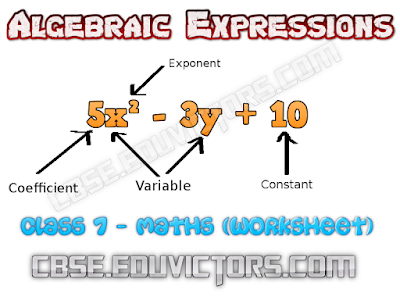## Lines and Angles (Worksheet)

Directions: Fill in the blanks to make the statements true.

1. If sum of measures of two angles is 90°, then the angles are _________.

3. If the sum of measures of two angles is 180°, then they are _________.

3. A transversal intersects two or more than two lines at _________ points.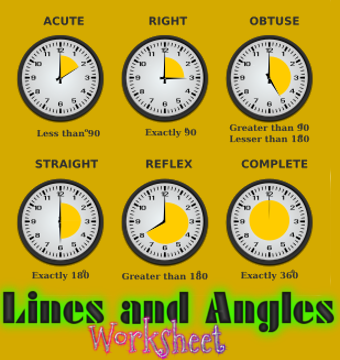## Divisibility Rules of Various Numbers

(2-25)

Divisibility by 2:
A number is divisible by 2 if its unit’s digit is even or 0.

Divisibility by 3:
A number is divisible by 3 if the sum of its digits are divisible by 3.

Divisibility by 4:
A number is divisible by 4 if the last 2 digits are divisible by 4, or if the last two digits are 0’s.

Divisibility by 5:
A number is divisible by 5 if its unit’s digit is 5 or 0.

Divisibility by 6:
A number is divisible by 6 if it is simultaneously divisible by 2 and 3.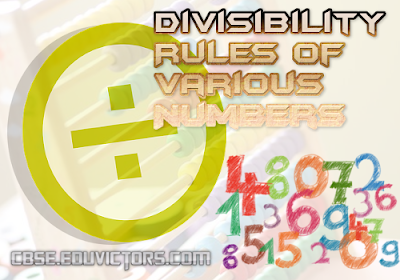## Exponents and Powers (MCQs)

Q1: Simplify 2³

(a) 6
(b) 8
(c) 10
(d) 222

Q2: What is the value of 3⁰?

(a) 0
(b) 1
(c) 3
(d) Not Defined

Q3: Value of 12² is _____

(a) 2
(b) 24
(c) 144
(d) 0## Mathematics - Fractions and Decimals - NCERT Exercise 2.6

Question 1: Find:
(i) 0.2 ✕ 6 (ii) 8 ✕ 4.6 (iii) 2.71 ✕ 5
(iv) 20.1 ✕ 43 (v) 0.05 ✕ 7 (vi) 211.02 ✕ 4
(vii) 2 ✕ 0.86

(i) 0.2 ✕ 6 = 1.2
(ii) 8 ✕ 4.6 = 36.8
(iii) 2.71 ✕ 5 = 13.55
(iv) 20.1 ✕ 4 = 80.4
(v) 0.05 ✕ 7 = 0.35
(vi) 211.02 ✕ 4 = 844.08
(vii) 2 ✕ 0.86 = 1.72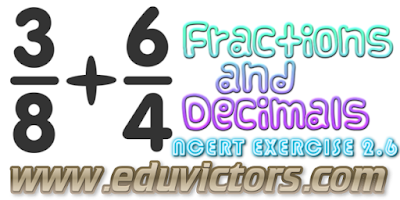## Chapter – 12 - Algebraic Expressions NCERT Exercise 12.1

Question 1: Get the algebraic expressions in the following cases using variables, constants and arithmetic operations:

(i) Subtraction of z from y .
(ii) One-half of the sum of numbers x and y .
(iii) The number z multiplied by itself.
(iv) One-fourth of the product of numbers p and q .
(v) Numbers x and y both squared and added.
(vi) Number 5 added to three times the product of m and n .
(vii) Product of numbers y and z subtracted from 10.
(viii) Sum of numbers a and b subtracted from their product.

(i) y - z

(ii)
(x+y)
-----
2

(iii)  z²

(iv)  pq
----
4

(v)   x² + y²

(vi) 3mn + 5

(vii) 10 - yz

(viii) ab - ( a + b )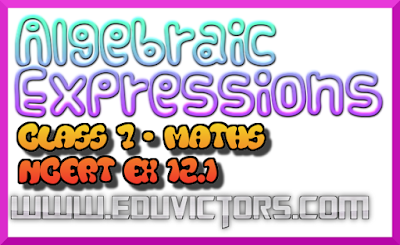## Chapter 2 - Decimals - Multiplication and Division (Worksheet)

Multiplying Decimals

Q1. Multiply the decimals.
4.4 ✕ 4.6
9.06 ✕ 54
4.23 ✕ 2.41
8.13 ✕ 3.6

Q2. Solve:
____ ✕ 53.99 = 539.9
87.13 ✕ ____ = 87130
10 ✕ _____ = 913
_____ ✕ 1000 = 85300
88.233 ✕ 100 = _____

Q3. Convert to fractions and multiply.
87.0 ✕ 5.2
34.10 ✕ 70.20## Class 7 - Maths - Algebraic Expressions - Practice Sheet

Q1. Classify as monomial, binomial and trinomial.

(a) x - x² + x³
(b) -26ab
(c) -7p²q -7pq²
(d) -27a² -125b²
(e) 2978

Q2. Form algebraic expressions from the following statements.

(a) Sum of the terms 5x and (-9y)
(b) Sum of the squares of 4 and x
(c) Product of a and b added to the sum of y and 20.
(d) Two times number 9 is subtracted from the product of y and z.